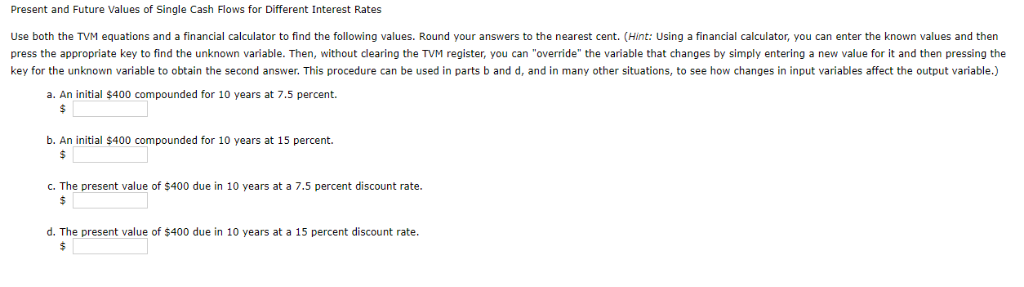Homework Help Question & Answers

# Present and Future Values of Single Cash Flows for Different Interest Rates Use both the TVM equations and a financial calculator to find the following values. Round your answers to the nearest cent....Present and Future Values of Single Cash Flows for Different Interest Rates Use both the TVM equations and a financial calculator to find the following values. Round your answers to the nearest cent. (Hint: Using a financial calculator, you can enter the known values and thern press the appropriate key to find the unknown variable. Then, without clearing the TVM register, you can "override" the variable that changes by simply entering a new value for it and then pressing the key for the unknown variable to obtain the second answer. This procedure can be used in parts b and d, and in many other situations, to see how changes in input variables affect the output variable.) a. An initial \$400 compounded for 10 years at 7.5 percent. b. An initial \$400 compounded for 10 years at 15 percent. c. The present value of \$400 due in 10 years at a 7.5 percent discount rate d. The present value of \$400 due in 10 years at a 15 percent discount rate.

a.Amount = \$400

Future Value = 400*(1.075)10 = \$824.41

b.Future Value = 400(1.15)10 = \$1,618.22

c.Present Value = 400/(1.075)10 = \$194.08

d.Present Value = 400/(1.15)10 = \$98.87

##### Add Answer of: Present and Future Values of Single Cash Flows for Different Interest Rates Use both the TVM equations and a financial calculator to find the following values. Round your answers to the nearest cent....
More Homework Help Questions Additional questions in this topic.

• #### If 3000 dollars is invested in a bank account at an interest rate of 6 per cent per year, find the amount in the bank after 12 years if interest is compounded annually Find the amount in the bank after 12 years if interest is compounded quaterly Find

Need Online Homework Help?

Get FREE EXPERT Answers
WITHIN MINUTES
Related Questions